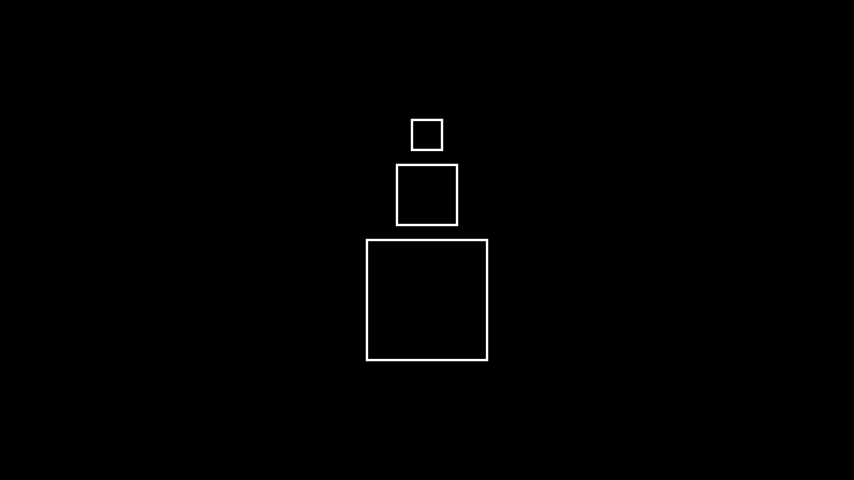# Square#

Qualified name: `manim.mobject.geometry.polygram.Square`

class Square(side_length=2.0, **kwargs)[source]#

Bases: `Rectangle`

A rectangle with equal side lengths.

Parameters
• side_length (float) – The length of the sides of the square.

• kwargs – Additional arguments to be passed to `Rectangle`.

Examples

Example: SquareExample```from manim import *

class SquareExample(Scene):
def construct(self):
square_1 = Square(side_length=2.0).shift(DOWN)
square_2 = Square(side_length=1.0).next_to(square_1, direction=UP)
square_3 = Square(side_length=0.5).next_to(square_2, direction=UP)
 `animate` Used to animate the application of any method of `self`. `animation_overrides` `color` `depth` The depth of the mobject. `fill_color` If there are multiple colors (for gradient) this returns the first one `height` The height of the mobject. `n_points_per_curve` `sheen_factor` `stroke_color` `width` The width of the mobject.